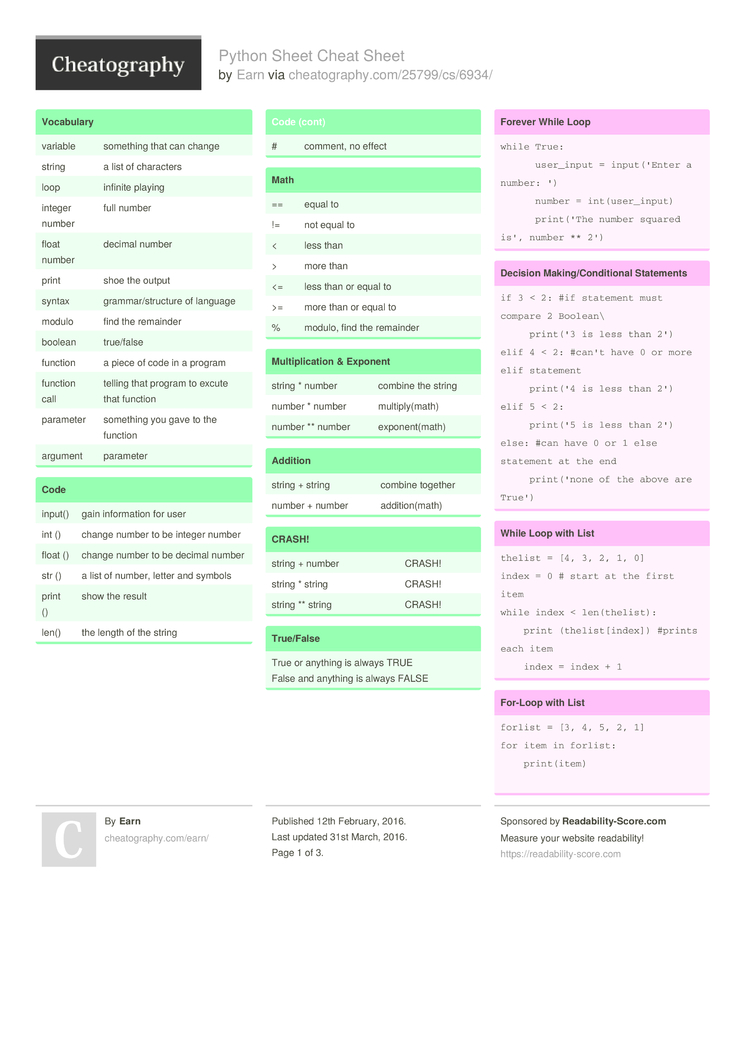# Python Sheet Cheat Sheet by Earn

### Vocabulary

 variable something that can change string a list of characters loop infinite playing integer number full number float number decimal number print shoe the output syntax gramma­r/s­tru­cture of language modulo find the remainder boolean true/false function a piece of code in a program function call telling that program to excute that function parameter something you gave to the function argument parameter

### Code

 input() gain inform­ation for user int () change number to be integer number float () change number to be decimal number str () a list of number, letter and symbols print () show the result len() the length of the string # comment, no effect

### Math

 == equal to != not equal to < less than > more than <= less than or equal to >= more than or equal to % modulo, find the remainder

### Multip­lic­ation & Exponent

 string * number combine the string number * number multip­ly(­math) number ** number expone­nt(­math)

 string + string combine together number + number additi­on(­math)

### CRASH!

 string + number CRASH! string * string CRASH! string ** string CRASH!

### True/False

 True or anything is always TRUE False and anything is always FALSE

### Forever While Loop

 ```while True:       user_input = input('Enter a number: ')       number = int(user_input)       print('The number squared is', number ** 2')```

### Decision Making­/Co­ndi­tional Statements

 ```if 3 < 2: #if statement must compare 2 Boolean\      print('3 is less than 2') elif 4 < 2: #can't have 0 or more elif statement      print('4 is less than 2') elif 5 < 2:      print('5 is less than 2') else: #can have 0 or 1 else statement at the end      print('none of the above are True')```

### While Loop with List

 ```thelist = [4, 3, 2, 1, 0] index = 0 # start at the first item while index < len(thelist):     print (thelist[index]) #prints each item     index = index + 1```

### For‐Loop with List

 ```forlist = [3, 4, 5, 2, 1] for item in forlist:     print(item)```

### Functions

 ```#function with no parameters/arguments #and no return value #return is optional if you do not return a value def nameOfFunction():     print (‘This function has no parameters’)     print (‘This function has no return value’)     return # no value, just exits the function                         ______________________________________ #function call nameOfFunction() #function with 1 parameter/argument def testFunction(param):     print (‘This function has 1 parameter’)     print (param) #function call testFunction (“this is the parameter value”) ______________________________________ #function with 2 parameters and a return value def function3(param1, param2):     print(‘This function has 2 parameters’)     return param1 + param2 # return value #function call and store the result in a variable returnValue = function3(2, 3) print (returnValue)```

### Count Even&Odd #

 ```#repeatly receives positive integers from user and exit when gets negative number, then count even and odd number evenCount = 0 oddCount = 0 while True:      user_input = int(input("Enter number: "))      if user_input < 0:          print("Total even: ",evenCount)          print("Total odd: ",oddCount)          break elif user_input > 0:      if user_input % 2 == 0:          evenCount = evenCount + 1      else:          oddCOunt = oddCount +1```

### Mystring

 ```' ' ' expected output: 0 01 012 0123 01234 ' ' ' mystring = " " count = 0 while count < 5:      mystring = mystring + str(count)      print(mrstring)      count = count + 1```

### Condition While Loop

 ```count = 0 #start at 0 while count < 10: #loop while count is less than 10        print(count) #will print number 0-9        count = count + 1 #must increase count ' ' ' output 0 to 9 ' ' '```

### Countdown Machine

 ```user_number = input("What number do you want to count down? ") number = int(user_number) countdown_string = ' ' while number > 0:       countdown_number = countdown_string + str(number) + " "       number = number - 1 #print(number) print(countdown_string)```

### Random List

 ```import random #create a list mylist = [-1,1.2,11,"srujryju","yikjghk"] random_item = random.choice(mylist) print(mylist, random_item)```

### Reverse Word

 ```while True:       word = input("Please enter a word")       index = 0       reverse = ' ' while int(index) < len(word):      reverse = word[index] + (reverse)      index = int(index) + 1 print ("Reverse: ", reverse)```

### Function pir*2

 ```def areaoscircle(r):       pi = 3.1415       return pi r * 2```

### Printing values

 print(­"­hel­lo", "­the­re") #displays hello there print(­"­hel­lo" + "­the­re") #displays hellothere

### Combining Strings (Conca­ten­ation)

 "­hi" + "­the­re" == "­hit­her­e" "­hi" * 5 == "­hih­ihi­hih­i"

### Naming Convention

 Rule for giving name - letter - numbers - unders­core_ Valid name - _mystr - my3 - Hello_­there Invalid name - 3my = "­hi" -- cannot start with number - first name = "­hi" - first-name - first+name

### Example

 Print(2) - integer Print(2.5) - floating point Print(­"­Hel­lo") - string Print(­mystr) - variable Print(­mystr, "­Hi",­2,1.0) -- commas mystr = "­Hi" mystr <- name "­Hi" <- value can change print(­int­(1.5)) -> 1 print(­int­("2")) -> 2 print(­flo­at(1)) -> 1.0 anything to a float

### Example Code

 mylist = [1, 'hello', 2.5] print (mylist) print (mylist2) print (mylist3) # how to make a list with all numbers from 0-99 mynumbers = range(5) for num on mynumbers: print (num)3 Pages
//media.cheatography.com/storage/thumb/earn_python-sheet.750.jpg

PDF (recommended)